Chapter 10 The `dplyr` Library

The `dplyr` (“dee-ply-er”) package is the preeminent tool for data wrangling in R (and perhaps, in data science more generally). It provides programmers with an intuitive vocabulary for executing data management and analysis tasks. Learning and utilizing this package will make your data preparation and management process faster and easier to understand. This chapter introduces the philosophy behind the library and an overview of how to use the library to work with dataframes using its expressive and efficient syntax.

10.1 A Grammar of Data Manipulation

Hadley Wickham, the creator of the `dplyr` package, fittingly refers to it as a Grammar of Data Manipulation. This is because the package provides a set of verbs (functions) to describe and perform common data preparation tasks. One of the core challenge in programming is mapping from questions about a dataset to specific programming operations. The presence of a data manipulation grammar makes this process smoother, as it enables you to use the same vocabulary to both ask questions and write your program. Specifically, the `dplyr` grammar lets you easily talk about and perform tasks such as:

• select specific features (columns) of interest from the data set
• filter out irrelevant data and only keep observations (rows) of interest
• mutate a data set by adding more features (columns)
• arrange the observations (rows) in a particular order
• summarize the data in terms of aspects such as the mean, median, or maximum
• join multiple data sets together into a single data frame

You can use these words when describing the algorithm or process for interrogating data, and then use `dplyr` to write code that will closely follow your “plain language” description because it uses functions and procedures that share the same language. Indeed, many real-world questions about a dataset come down to isolating specific rows/columns of the data set as the “elements of interest”, and then performing a simple comparison or computation (mean, count, max, etc.). While it is possible to perform this computation with basic R functions—the `dplyr` library makes it much easier to write and read such code.

10.2 Using `dplyr` Functions

The `dplyr` package provides functions that mirror the above verbs. Using this package’s functions will allow you to quickly and effectively write code to ask questions of your data sets.

Since `dplyr` is an external package, you will need to install it (once per machine) and load it to make the functions available:

``````install.packages("dplyr")  # once per machine
library("dplyr")``````

After loading the library, you can call any of the functions just as if they were the built-in functions you’ve come to know and love.

For each `dplyr` function discussed here, the first argument to the function is a data frame to manipulate, with the rest of the arguments providing more details about the manipulation.

IMPORTANT NOTE: inside the function argument list (inside the parentheses), we refer to data frame columns without quotation marks—that is, we just give the column names as variable names, rather than as character strings. This is refered to as non-standard evaluation, and is described in more detail below; while it makes code easier to write and read, it can occasionally create challenges.

The images in this section come from the RStudio’s STRATA NYC R-Day workshop, which was presented by Nathan Stephens.

10.2.1 Select

The `select()` operation allows you to choose and extract columns of interest from your data frame.

``````# Select `storm` and `pressure` columns from `storms` data frame
storm_info <- select(storms, storm, pressure)``````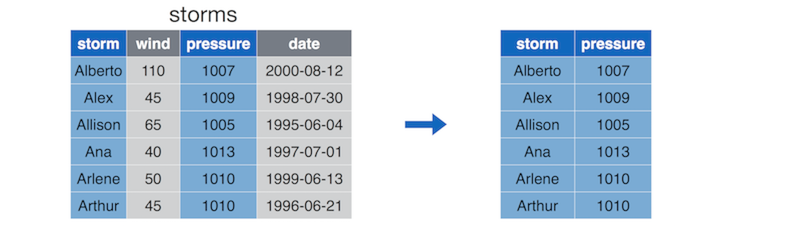Diagram of the `select()` function (by Nathan Stephens).

The `select()` function takes in the data frame to select from, followed by the names of the columns you wish to select (quotation marks are optional!)

This function is equivalent to simply extracting the columns:

``````# Extract columns by name
storm_info <- storms[, c("storm", "pressure")]  # Note the comma!``````

But easier to read and write!

10.2.2 Filter

The `filter()` operation allows you to choose and extract rows of interest from your data frame (contrasted with `select()` which extracts columns).

``````# Select rows whose `wind` column is greater than or equal to 50
some_storms <- filter(storms, wind >= 50)``````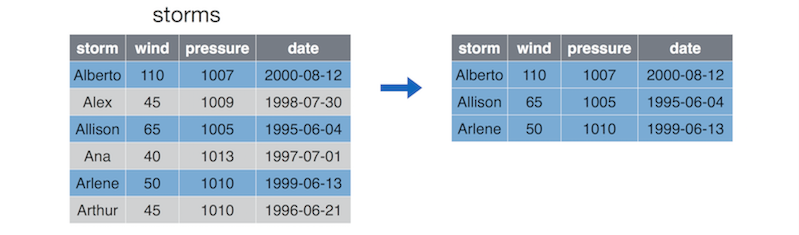Diagram of the `filter()` function (by Nathan Stephens).

The `filter()` function takes in the data frame to filter, followed by a comma-separated list of conditions that each returned row must satisfy. Note again that columns are provided without quotation marks!

• R will extract the rows that match all conditions. Thus you are specifying that you want to filter down a data frame to contain only the rows that meet Condition 1 and Condition 2.

This function is equivalent to simply extracting the rows:

``````# Extract rows by condition
some_storms <- storms[storms\$wind >= 50, ]  # Note the comma!``````

As the number of conditions increases, it is far easier to read and write `filter()` functions, rather than squeeze your conditions into brackets.

10.2.3 Mutate

The `mutate()` operation allows you to create additional columns for your data frame.

``````# Add `ratio` column that is ratio between pressure and wind
storms <- mutate(storms, ratio = pressure/wind)  # Replace existing `storms` frame with mutated one!``````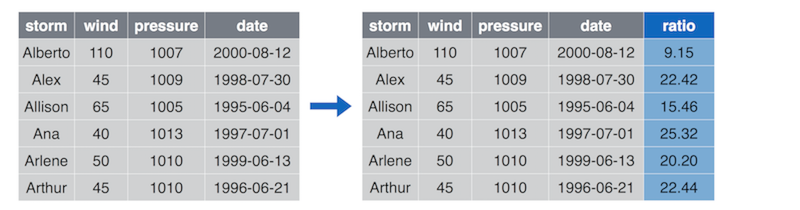Diagram of the `mutate()` function (by Nathan Stephens).

The `mutate()` function takes in the data frame to mutate, followed by a comma-separated list of columns to create using the same `name = vector` syntax you used when creating lists or data frames from scratch. As always, the names of the columns in the data frame are used without quotation marks.

• Despite the name, the `mutate()` function doesn’t actually change the data frame; instead it returns a new data frame that has the extra columns added. You will often want to replace the old data frame variable with this new value.

In cases where you are creating multiple columns (and therefore writing really long code instructions), you should break the single statement into multiple lines for readability. Because you haven’t closed the parentheses on the function arguments, R will not treat each line as a separate statement.

``````# Generic mutate command
more_columns <- mutate(
my_data_frame,
new_column_1 = old_column * 2,
new_column_2 = old_column * 3,
new_column_3 = old_column * 4
)``````

10.2.4 Arrange

The `arrange()` operation allows you to sort the rows of your data frame by some feature (column value).

``````# Arrange storms by INCREASING order of the `wind` column
sorted_storms <- arrange(storms, wind)``````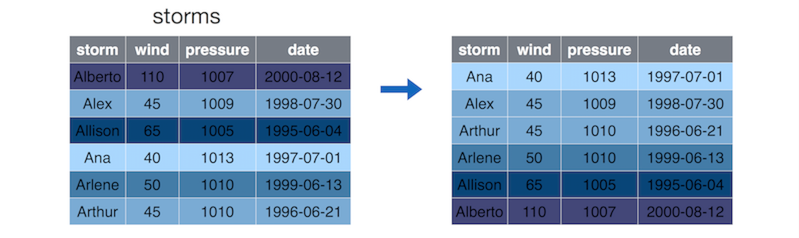Diagram of the `arrange()` function (by Nathan Stephens).

By default, the `arrange()` function will sort rows in increasing order. To sort in reverse (decreasing) order, place a minus sign (`-`) in front of the column name (e.g., `-wind`). You can also use the `desc()` helper function (e.g, `desc(wind)`).

• You can pass multiple arguments into the `arrange()` function in order to sort first by `argument_1`, then by `argument_2`, and so on.

• Again, this doesn’t actually modify the argument data frame—instead returning a new data frame you’ll need to store.

10.2.5 Summarize

The `summarize()` function (equivalently `summarise()` for those using the British spelling) will generate a new data frame that contains a “summary” of a column, computing a single value from the multiple elements in that column.

``````# Compute the median value of the `amount` column
summary <- summarize(pollution, median = median(amount))``````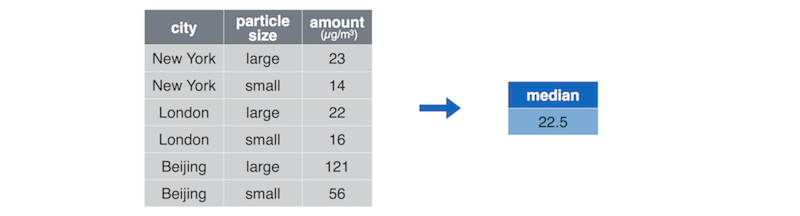Diagram of the `summarize()` function (by Nathan Stephens).

The `summarize()` function takes in the data frame to mutate, followed by the values that will be included in the resulting summary table. You can use multiple arguments to include multiple summaries in the same statement:

``````# Compute statistics for the `amount` column
summaries <- summarize(
pollution,
median = median(amount), # median value
mean = mean(amount), # "average" value
sum = sum(amount), # total value
count = n() # number of values (neat trick!)
)``````

Note that the `summarize()` function is particularly useful for grouped operations (see below), as you can produce summaries of different groups of data.

10.2.6 Distinct

The `distinct()` operation allows you to extract distinct values (rows) from your data frame—that is, you’ll get one row for each different value in the dataframe (or set of selected columns). This is a useful tool to confirm that you don’t have duplicate observations, which often occurs in messy datasets.

For example (no diagram available):

``````# Create a quick data frame
x <- c(1, 1, 2, 2, 3, 3, 4, 4)  # duplicate x values
y <- 1:8                        # unique y values
my_df <- data.frame(x, y)

# Select distinct rows, judging by the `x` column
distinct_rows <- distinct(my_df, x)
#   x
# 1 1
# 2 2
# 3 3
# 4 4

# Select distinct rows, judging by the `x` and `y`columns
distinct_rows <- distinct(my_df, x, y)  # returns whole table, since no duplicate rows``````

While this is a simple way to get a unique set of rows, be careful not to lazily remove rows of your data which may be important.

10.3 Multiple Operations

You’ve likely encountered a number of instances in which you want to take the results from one function and pass them into another function. Your approach thus far has often been to create temporary variables for use in your analysis. For example, if you’re using the `mtcars` dataset, you may want to ask a simple question like,

Which 4-cylinder car gets the best milage per gallon?

This simple question actually requires a few steps:

1. Filter down the dataset to only 4-cylinder cars
2. Of the 4-cylinder cars, filter down to the one with the highest mpg
3. Select the car name of the car

You could then implement each step as follows:

``````# Preparation: add a column that is the car name
mtcars_named <- mutate(mtcars, car_name = row.names(mtcars))

# 1. Filter down to only four cylinder cars
four_cyl <- filter(mtcars_named, cyl == 4)

# 2. Filter down to the one with the highest mpg
best_four_cyl <- filter(four_cyl, mpg == max(mpg))

# 3. Select the car name of the car
best_car_name <- select(best_four_cyl, car_name)``````

While this works fine, it clutters the work environment with variables you won’t need to use again, and which can potentially step on one another’s toes. It can help with readability (the results of each step is explicit), but those extra variables make it harder to modify and change the algorithm later (you have to change them in two places).

An alternative to saving each step as a distinct, named variable would be to utilize anonymous variables and write the desired statements nested within other functions. For example, you could write the algorithm above as follows:

``````# Preparation: add a column that is the car name
mtcars_named <- mutate(mtcars, car_name = row.names(mtcars))

# Write a nested operation to return the best car name
best_car_name <- select( # 3. Select car name of the car
filter( # 2. Filter down to the one with the highest mpg
filter( # 1. Filter down to only four cylinder cars
mtcars_named, # arguments for the Step 1 filter
cyl == 4
),
mpg == max(mpg) # other arguments for the Step 2 filter
),
car_name # other arguments for the Step 3 select
)``````

This version uses anonymous variables—result values which are not assigned to names (so are anonymous), but instead are immediately used as the arguments to another function. You’ve used these frequently with the `print()` function and with filters (those vectors of `TRUE` and `FALSE` values)—and even the `max(mpg)` in the Step 2 filter is an anonymous variable!

This nested version performs the same results as the temporary variable version without creating the extra variables, but even with only 3 steps it can get quite complicated to read—in a large part because you have to think about it “inside out”, with the stuff in the middle evaluating first. This will obviously become undecipherable for more involved operations.

10.3.1 The Pipe Operator

Luckily, `dplyr` provides a cleaner and more effective way of achieving the same task (that is, using the result of one function as an argument to the next). The pipe operator (`%>%`) indicates that the result from the first function operand should be passed in as the first argument to the next function operand!

As a simple example:

``````# nested version: evaluate c(), then max(), then print()
print(max(c(2, 0, 1)))

# pipe version
c(1,2,3) %>%   # do first function
max() %>%   # which becomes the _first_ argument to the next function
print()  # which becomes the _first_ argument to the next function``````

Or as another version of the above data wrangling:

``````# Preparation: add a column that is the car name
mtcars_named <- mutate(mtcars, car_name = row.names(mtcars))

best_car_name <- filter(mtcars_named, cyl == 4) %>% # Step 1
filter(mpg == max(mpg)) %>% # Step 2
select(car_name) # Step 3``````
• Yes, the `%>%` operator is awkward to type and takes some getting use to (especially compared to the command-line’s use of `|` to pipe). However, you can ease the typing by using the RStudio keyboard shortcut `cmd + shift + m`.

The pipe operator is part of the `dplyr` package (it is only available if you load that package), but it will work with any function, not just `dplyr` ones! This syntax, while slightly odd, can completely change and simplify the way you write code to ask questions about your data!

10.4 Grouped Operations

`dplyr` functions are powerful, but they are truly awesome when you can apply them to groups of rows within a data set. For example, the above use of `summarize()` isn’t particularly useful since it just gives a single summary for a given column (which you could have done anyway). However, a grouped operation would allow you to compute the same summary measure (`mean`, `median`, `sum`, etc.) automatically for multiple groups of rows, enabling you to ask more nuanced questions about your data set.

The `group_by()` operation allows you to break a data frame down into groups of rows, which can then have the other verbs (e.g., `summarize`, `filter`, etc). applied to each one.

``````# Get summary statistics by city
city_summary <- group_by(pollution, city) %>%
summarize( # first argument (the data frame) is received from the pipe
mean = mean(amount),
sum = sum(amount),
n = n()
)``````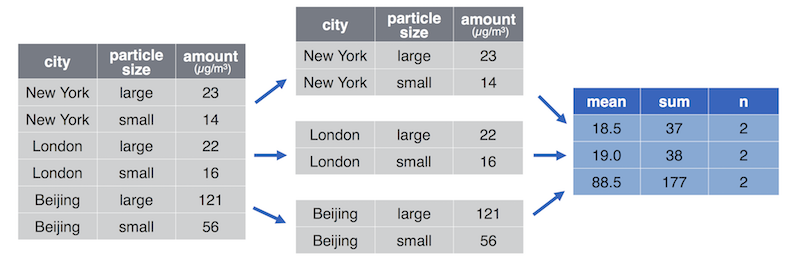Diagram of the `group_by()` function (by Nathan Stephens).

As another example, if you were using the `mtcars` dataset, you may want to answer this question:

What are the differences in mean miles per gallon for cars with different numbers of gears (3, 4, or 5)?

This simple question requires computing the mean for different subsets of the data. Rather than explicitly breaking your data into different groups (a.k.a. bins or chunks) and running the same operations on each, you can use the `group_by()` function to accomplish this in a single command:

``````# Group cars by gear number, then compute the mean and median mpg
gear_summary <- group_by(mtcars, gear) %>%  # group by gear
summarize(mean = mean(mpg))  # calculate mean
# Computing the difference between scores is done elsewhere (or by hand!)``````

Thus grouping can allow you to quickly and easily compare different subsets of your data!

10.5 Joins

When working with real-world data, you’ll often find that that data is stored across multiple files or data frames. This can be done for a number of reasons. For one, it can help to reduce memory usage (in the same manner as factors). For example, if you had a data frame containing information on students enrolled in university courses, you might store information about each course (the instructor, meeting time, and classroom) in a separate data frame rather than duplicating that information for every student that takes the same course. You also may simply want to keep your information organized: e.g., have student information in one file, and course information in another.

• This separation and organization of data is a core concern in the design of relational databases, a common topic of study within Information Schools.

But at some point, you’ll want to access information from both data sets (e.g., you need to figure out a student’s schedule), and thus need a way to combine the data frames. This process is called a join (because you are “joining” the data frames together). When you perform a join, you identify columns which are present in both tables. Those column values are then used as identifiers to determine which rows in each table correspond to one another, and thus will be combined into a row in the resulting joined table.

The `left_join()` operation is one example of a join. This operation looks for matching columns between the two data frames, and then returns a new data frame that is the first (“left”) operand with extra columns from the second operand added on.

``````# Combine (join) songs and artists data frames
left_join(songs, artists)``````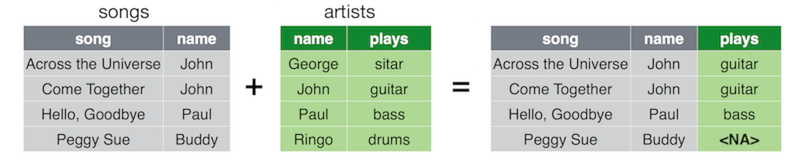Diagram of the `left_join()` function (by Nathan Stephens).

To understand how this works, consider a specific example where you have a table of student_ids and the students’ contact information. You also have a separate table of student_ids and the students’ majors (your institution very well may store this information in separate tables for privacy or organizational reasons).

``````# Table of contact information
student_contact <- data.frame(
student_id = c(1, 2, 3, 4), # id numbers
email = c("id1@school.edu", "id2@school.edu", "id3@school.edu", "id4@school.edu")
)

# Table of information about majors
student_majors <- data.frame(
student_id = c(1, 2, 3), # id numbers
major = c("sociology", "math", "biology")
)``````

Notice that both tables have a `student_id` column, allowing you to “match” the rows from the `student_contact` table to the `student_majors` table and merge them together:

``````# Join tables by the student_id column
merged_student_info <- left_join(student_contact, student_majors)
#    student_id          email     major
# 1          1 id1@school.edu sociology
# 2          2 id2@school.edu      math
# 3          3 id3@school.edu   biology
# 4          4  id4@school.edu      <NA>``````

When you perform this left join, R goes through each row in the table on the “left” (the first argument), looking at the shared column(s) (`student_id`). For each row, it looks for a corresponding value in `student_majors\$student_id`, and if it finds one then it adds any data from columns that are in `student_majors` but not in `student_contact` (e.g., `major`) to new columns in the resulting table, with values from whatever the matching row was. Thus student #1 is given a `major` of “sociology”, student #2 is given a `major` of “math”, and student #4 is given a `major` of `NA` (because that student had no corresponding row in `student_majors`!)

• In short, a left join returns all of the rows from the first table, with all of the columns from both tables.

R will join tables by any and all shared columns. However, if the names of your columns don’t match specifically, you can also specify a `by` argument indicating which columns should be used for the matching:

``````# Use the named `by` argument to specify (a vector of) columns to match on
left_join(student_contact, student_majors, by="student_id")``````
• With the `by` argument, column name is a string (in quotes) because you’re specifying a vector of column names (the string literal is a vector length 1).

Notice that because of how a left join is defined, the argument order matters! The resulting table only has rows for elements in the left (first) table; any unmatched elements in the second table are lost. If you switch the order of the operands, you would only have information for students with majors:

``````# Join tables by the student_id column
merged_student_info <- left_join(student_majors, student_contact)  # switched order!
#   student_id     major          email
# 1          1 sociology id1@school.edu
# 2          2      math id2@school.edu
# 3          3   biology id3@school.edu``````

You don’t get any information for student #4, because they didn’t have a record in the left-hand table!

Because of this behavior, `dplyr` (and relational database systems in general) provide a number of different kinds of joins, each of which influences which rows are included in the final table. Note that in any case, all columns from both tables will be included, with rows taking on any values from their matches in the second table.

• `left_join` All rows from the first (left) data frame are returned. That is, you get all the data from the left-hand table, with extra column values added from the right-hand table. Left-hand rows without a match will have `NA` in the right-hand columns.

• `right_join` All rows from the second (right) data frame are returned. That is, you get all the data from the right-hand table, with extra column values added from the left-hand table. Right-hand rows without a match will have `NA` in the left-hand columns. This is the “opposite” of a `left_join`, and the equivalent of switching the operands.

• `inner_join` Only rows in both data frames are returned. That is, you get any rows that had matching observations in both tables, with the column values from both tables. There will be no additional `NA` values created by the join. Observations from the left that had no match in the right, or observations in the right that had no match in the left, will not be returned at all.

• `full_join` All rows from both data frames are returned. That is, you get a row for any observation, whether or not it matched. If it happened to match, it will have values from both tables in that row. Observations without a match will have `NA` in the columns from the other table.

The key to deciding between these is to think about what set of data you want as your set of observations (rows), and which columns you’d be okay with being `NA` if a record is missing.

Note that these are all mutating joins, which add columns from one table to another. `dplyr` also provides filtering joins which exclude rows based on whether they have a matching observation in another table, and set operations which combine observations as if they were set elements. See the documentation for more detail on these options, but in this course we’ll be primarily focusing on the mutating joins described above.

10.6 Non-Standard Evaluation vs. Standard Evaluation

One of the features that makes `dplyr` such a clean and attractive way to write code is that inside of each function, you’ve been able to write column variable names without quotes. This is called non-standard evaluation (NSE) (it is not the standard way that code is evaluated, or interpreted), and is useful primarily because of how it reduces typing (along with some other benefits when working with databases). In particular, `dplyr` will “quote” expressions for you, converting those variables (symbols) into values that can be used to refer to column names.

Most of the time this won’t cause you any problems—you can either use NSE to refer to column names without quotes, or provide the quotes yourself. You can even use variables to store the name of a column of interest!

``````# Normal, non-standard evaluation version
mpg <- select(mtcars, mpg)

# "Standard-evaluation" version (same result)
mpg <- select(mtcars, "mpg") # with quotes! "mpg" is a normal value!

# Make the column name a variable
which_col <- "mpg"
my_column <- select(mtcars, which_col)``````

However, this NSE can sometimes trip you up when using more complex functions such as `summarize()` or `group_by()`, or when you want to create your own functions that use NSE.

``````which_col <- "mpg"
summarize(mtcars, avg = mean(which_col))  # In mean.default(which_col) :
# argument is not numeric or logical: returning NA``````

In this case, the `summarize()` function is trying to “quote” what we typed in (the `which_col` variable name&dmash;not it’s `mpg` value), and then hitting a problem because there is no column of that name (it can’t resolve that column name to a column index).

To fix this problem, there are two parts: first, you need to explicitly tell R that the value of `which_col` (`mpg`) is actually the value that needs to be automatically “quoted”—that is, that `mpg` is really a variable! Variable names in R are referred to as symbols—a symbol refers to the variable label itself. You can explicitly change a value into a symbol by using the `rlang::sym()` function (the `sym()` function found in the `rlang` library; the `::` indicates that the function belongs to a library).

``````which_col_sym <- rlang::sym(which_col)  # convert to a symbol
print(which_col_sym)  # => mpg (but not in quotes, because it's not a string!)``````

Second, you will need to tell the `summarize()` function that it should not quote this symbol (because you’ve already converted it into a variable)—what is called unquoting. In `dplyr`, you “unquote” a parameter to a method by including two exclamation points in front of it:

``summarize(mtcars, avg = mean(!!which_col_sym))  # arranges by the specified column``

There are many more details involved in this “quoting/unquoting” process, which are described in this tutorial (though that is currently being updated with better examples).

10.6.1 Explicit Standard Evaluation

Alternatively, older versions of `dplyr` supplied functions that explicitly performed standard evaluation (SE)—that is, they provide no quoting and expected you to do that work yourself. While now considered deprecated, they can still be useful if you are having problems with the new quoting system. These functions have the exact same names as the normal verb functions, except are followed by an underscore (`_`):

``````# Normal, non-standard evaluation version
mpg <- select(mtcars, mpg)

# Standard-evaluation version (same result)
mpg <- select_(mtcars, 'mpg')  # with quotes! 'mpg' is a normal value!

# Normal, non-standard evaluation version of equations
mean_mpg <- summarize(mtcars, mean(mpg))

# Standard-evaluation version of equations (same result)
mean_mpg <- summarize_(mtcars, 'mean(mpg)')

# Which column you're interested in
which_column <- 'mpg'

# Use standard evaluation to execute function:
my_column <- arrange_(mtcars, which_column)``````

Yes, it does feel a bit off that the “normal” way of using `dplyr` is the “non-standard” way. Remember that using SE is the “different” approach

The non-standard evaluation offered by `dplyr` can make it quick and easy to work with data when you know its structure and variable names, but can be a challenge when trying to work with variables. Often in that case, you may want to instead use the standard data frame syntax (e.g., bracket notation) described in Chapter 9.